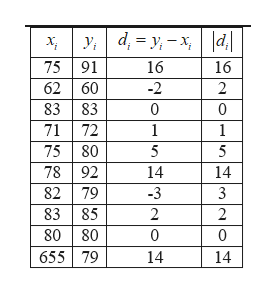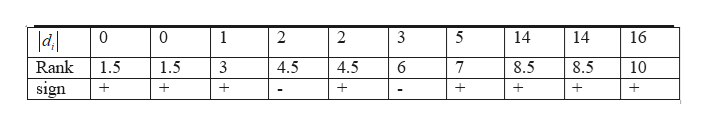# The results of a pre-test and a post-test for ten students is given below. Is there a significant difference in the median score difference post-test-pre-test? Test with the Wilcoxon Signed-Rank Test with a 5% significance level. You may assume the distribution of differences is symmetric.Pre-Test            75  62  83  71  75  78  82  83  80  65Post-Test          91  60  83  72  80  92  79  85  80  79

Question
26 views

The results of a pre-test and a post-test for ten students is given below. Is there a significant difference in the median score difference post-test-pre-test? Test with the Wilcoxon Signed-Rank Test with a 5% significance level. You may assume the distribution of differences is symmetric.

Pre-Test            75  62  83  71  75  78  82  83  80  65

Post-Test          91  60  83  72  80  92  79  85  80  79

check_circle

Step 1

Denote µd as the median difference for post and pre-test.

Hypotheses:

Null hypothesis: The median difference is zero.

Alternative hypothesis: The median difference differs from zero.

It is given that the distribution of difference is symmetric. Therefore, Wilcoxon-signed rank test can be used here.

The necessary calculations for finding test statistic is given below:help_outlineImage Transcriptioncloseу, а, - у, — х, || 75 91 62 60 83 83 71 72 75 80 78 92 82 79 83 85 80 80 655 79 16 16 -2 2 0 0 1 1 5 5 14 14 -3 2 2 0 14 14 fullscreen
Step 2

The rank table is given below:help_outlineImage Transcriptionclose0 0 1 2 2 3 5 14 14 16 Rank 1.5 1.5 3 4.5 4.5 6 7 8.5 8.5 10 sign + + + fullscreen
Step 3

The sum of positive ranks is denoted as W+.

The sum of negative ranks is denoted as W-.

From the above table W+=1.5 + 1.5 + 3 + 4.5 + 7 + 8.5 + 8.5 + 10 = 44.5

W- = 4.5 + 6 = 10.5

Test stat...

### Want to see the full answer?

See Solution

#### Want to see this answer and more?

Solutions are written by subject experts who are available 24/7. Questions are typically answered within 1 hour.*

See Solution
*Response times may vary by subject and question.
Tagged in

### Hypothesis Testing